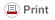# QUERI – Quality Enhancement Research Initiative

| View All QI Methods | Download QI Handbook |## 27. Modeling and Optimization

a. Definition: Many processes can be described in mathematical terms using a set of equations, perhaps also using a computer program. These models can be useful to gain insight as to how a process works and cans be used to determine an improvement or optimum design. Such mathematical models are used effectively in many industries, including healthcare and research. The models describe how a process functions, including relationships between its parts and constraints. Some models find an optimum design, whereas others are merely descriptive but provide insights for improvement. Simulation (see Section 42) is an Example of a descriptive and helpful model but which does not necessarily find an optimum. Other popular mathematical modeling strategies used in healthcare include:

• Linear Programming: Attributes of a system are described as a group of variables (numerical values). Linear (straight line) equations describe the relationships and constraints for the variables of the system being modeled. An objective function equation includes the aspects of the system that are desirable and their relative importance. A solution is the point where the variables will produce the optimum result for the objective function.
• Integer Programming: Similar to Linear Programming but where the attributes of the system are integers. Often systems have variables which can only be whole numbers such as a number of appointments or a number of patients.
• Markov Chains: A stochastic model where the future state depends only on the current state. Such a model means that the future does not depend on the past. It makes feasible certain calculations such as weather forecasting or to model a series of clinical decisions.
• Queuing Models: Various aspects of waiting lines can be determined based on elements of a queuing system such as arrival rates, service times and number of servers. These models assume randomness but with definable probability distributions. The time a patient will likely spend in a clinic's waiting room can be calculated, for Example.
• Forecasting models: several different mathematical models can be used to extrapolate past history to predict the future or to use a variety of data to forecast events. This can be used for inventory planning or staffing based on expected future demand.

b. Literature: There is considerable literature about each type of modeling approach within the field of applied mathematics as well as computer software to create and analyze such models. Operations Research refers to this field of using advanced analytic methods for decision making and is sometimes referred to as Decision Science or Management Science or Data Analytics. The professional societies of Operations Research include considerable publications related to healthcare.

• The Institute for Operations Research and the Management Sciences (INFORMS) publishes multiple scholarly journals about operations research. Also, there are a variety of journals on applied mathematics in healthcare, such as Operations Research for Health Care, ISSN: 2211-6923, Elsevier Publishing.
• The Mayo Clinic Robert D. and Patricia E. Kern Center for the Science of Health Care Delivery holds conferences regarding operations research and healthcare and is a source for publications in the field.
• Rais, Abdur, and Ana Viana. "Operations Research in Healthcare: a survey." International Transactions in Operational Research 18.1 (2011): 1-31.

c. Example: A research project is considering multiple patient screening measures of which each measure has differing outcomes and costs. Linear programming can be used to find the optimum mix of measures. The model consists of several equations. The objective function for the model is an equation of the sum of the several outcome measures and their relative importance. There are constraints regarding available funds and constraints regarding outcome measures. A minimum reduction in readmissions and that certain clinical outcomes must exceed specified values are written as constraint equations. The solution of the equations is the point where the net total effect of the outcome measures, which is the objective function, is maximized but the mix of screening measures remains within the constraints. Thus, a research project would seek to determine all the elements of the linear programming model so that the solution can be found and relied upon.

d. Steps:

1) Develop a detailed understanding of a problem.

2) Identify the best modeling approach to represent the problem accurately. If a true optimization is needed then a descriptive model will not be sufficient. Sometimes using more than one approach is necessary.

3) Identify attributes of the system being modeled

4) Create a mathematical representation, in a computer if necessary and possible

5) Calculate the optimum, if that sort of model is created, or use insights from the model to create improvements